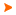Podaci o VU Početna stranicaArhiva nastavnih programaArhiva ak. kalendaraFinancial Mathematics
 Abbreviation: Load: 45(L) + 0(E) + 0(LE) + 0(CE)
 Lecturers in charge: Doc. dr. sc. Igor Velčić Lecturers: dr. sc. Petra Posedel ( Lectures ) Course description: The course introduces the concepts of both compounded and continuosly compounded interest, risk-free assets, term structure of interest rates and fixed income securities. The basics from stochastic calculus as a necessary instrument for quantitative analysis of financial markets, investment strategies, the concept of arbitrage and the funadamental theorem of finance are provided. Optimality in the sense of maximazing the rate of return, minimizing the variance in the rate of return and minimizing risk to the investor are explored. Effects of correlated movements in the values of investments are modelled and the Capital Asset Pricing Model (CAPM) is introduced. Models describing the price dynamics in both discrete time as the binomial model and continuous time as the model leading to the Black-Scholes formula are analyzed. The basics from option pricing and hedging are introduced.
 Lecture languages: - - -
 Compulsory literature: 2. Arbitrage Theory in Continuous Time;Thomas Björk ;Oxford University Press;1998
 Recommended literature: 1. Introduction to Mathematical Finance: Discrete-Time Models;S. R. Pliska;Blackwell Publishers;1997 3. Mathematics for Finance: An Introduction to Financial Engineering;M. Capiński and T. Zastawniak;Springer-Verlag;2003 4. An Undergraduate Introduction to Financial Mathematics;. Robert Buchanan;World Scientific Publishing Co. Pte. Ltd.;2006Legend L - Lectures E - Exercises LE - Laboratory exercises CE - Project laboratory * - Not graded| Početna stranica | Visoka učilišta | Korisničke stranice | | Na vrh | Copyright (c) 2006. Ministarstva znanosti, obrazovanja i športa. Sva prava zadržana. Programska podrška (c) 2006. Fakultet elektrotehnike i računarstva. Oblikovanje(c) 2006. Listopad Web Studio. Posljednja izmjena 2014-01-27